# Team:NCTU Formosa/Disease Occurrence Model

NCTU_Formosa: Disease Occurrence Model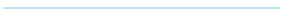###### Prediction Modeling

In order to cure the fungal diseases in reality, we used the method called Convolutional Neural Network(CNN) to catch the weather patterns that was hard to be recognized by humans. With this disease occurrence model, we can get the daily possibility of disease occurrences. Also, we set up a warning and auto-spraying system by combining the model with the IoTtalk to apply peptides into farmlands.

1. Datasets--the past fungal diseases data and the weather data
2. Convolutional Neural Network
3. Softmax function
4. How to define our model cost function and the optimizer
5. Connection to the IoTtalk systemFor the disease occurrence prediction modeling, NCTU_Formosa built a model predicting the diseases relating to everyday weather information that was based on the neural network. There were two kinds of data in our predict system, which were the recorded diseases happening data that were collected from the government agency and the weather data from the Central Meteorological Bureau's website that corresponded to the data forward. Then, we combined these two and then deleted the recorded data that had no weather data to match with.

Finally, our data contain weather feature and the Label. The weather feature is a two-dimensional array which has 14 days x 11 features. The 11 features include relative humidity, rainfall and the maximum, minimum, average of the temperature and air pressure. The Label contains two classes, negative(diseases that would not happen) and positive(diseases that would happen).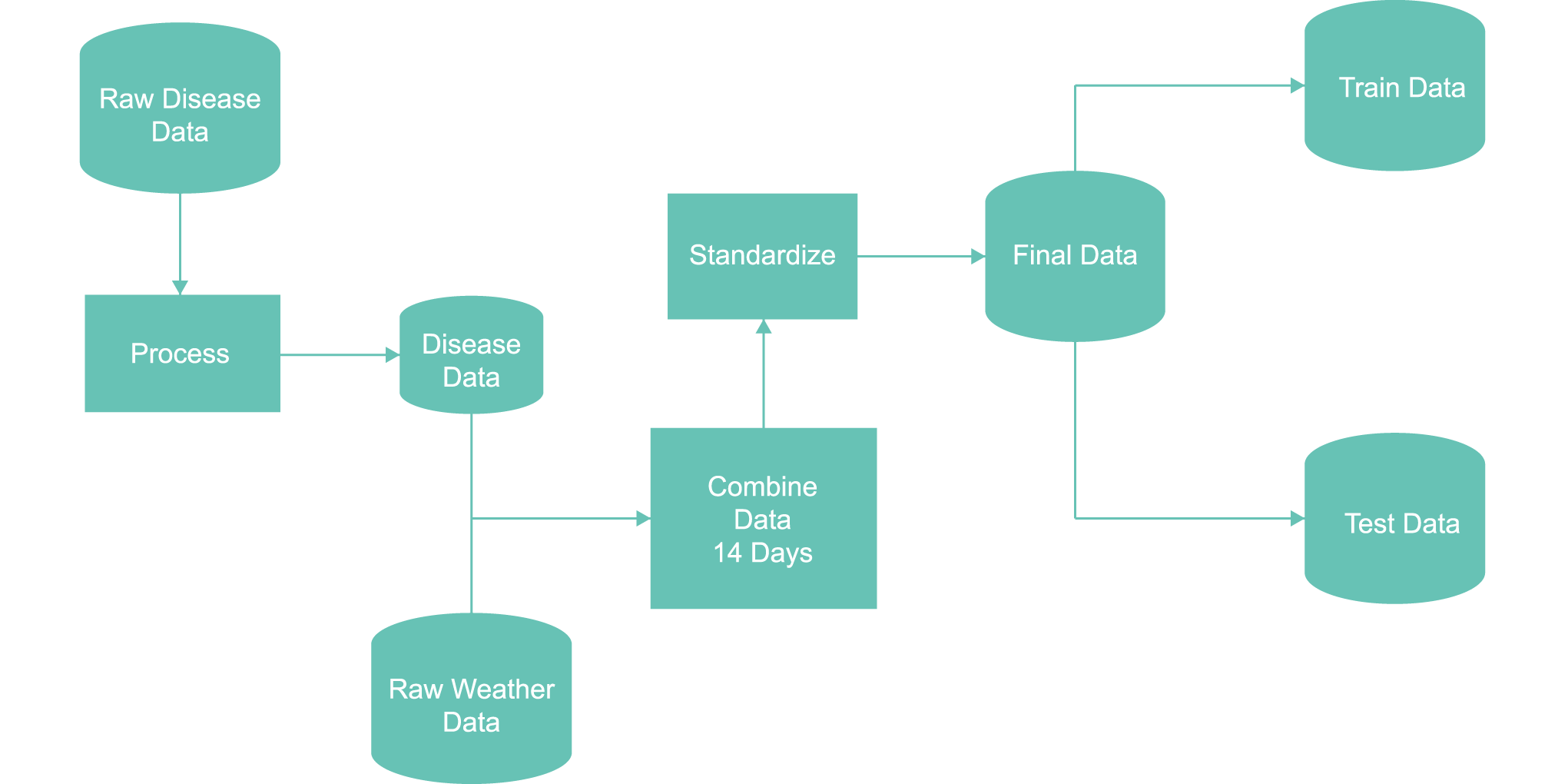#### Figure 1: Data process flow chart

In reality, the spore germination needs some specific weather conditions. Also, the plants may be influenced by the change of weather and become weak. However, this relationship is hard to be recognized by humans. Therefore, we used Convolutional Neural Network(CNN) as our method to catch the weather patterns.

# Convolutional Neural Network(CNN)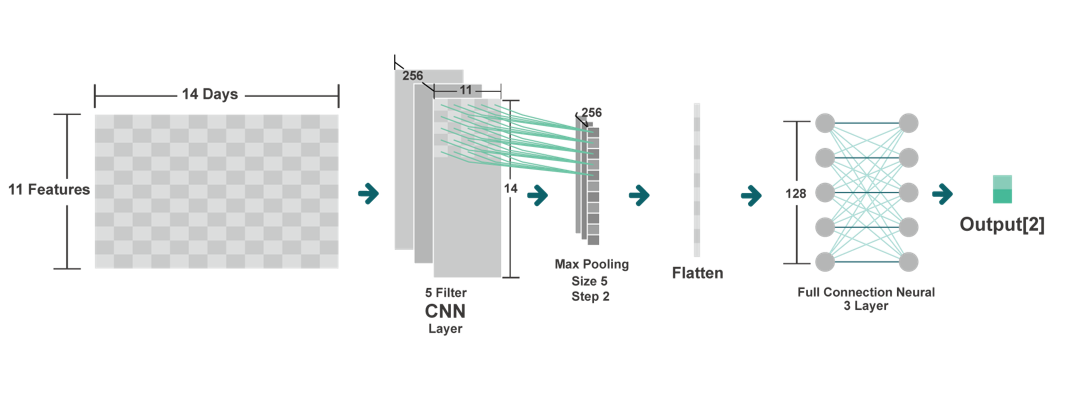#### Figure 2: This is an overview of our model,it contains the convolution layer, max pooling layer and multi full connection layer.

Convolutional Neural Network(CNN) is a powerful network that can automatically recognize the patterns of the features in a period of time, just like the favorite weather change to the fungal diseases in the life cycle. In other words, it means it can recognize the pathogen life cycle in a specific time and mark them out because life cycle’s patterns can happen everywhere over a period of time. For example, our model used weather data for the past two weeks as the model input, and the weather patterns can be found in this 14-day data.

After the CNN layer, the weather features then converted to weather change features, then a max pooling layer was added to filter some noises after the CNN layer. Weather patterns that caused diseases wouldn’t change in a short time, so the function of max pooling was to only return the maximum values in the filter.

$$f(x_i) = max(x_i)$$

For example: if the input array is [2,5,1,7,0,4] and the max pooling filter size is 2. When the filter step(distance the filter moves) is 1, the first max pooling output will be max(2,5)=5, and the second output is max(5,1) =5 and so on.

Because the max pooling output is the two-dimensional tensor, we will flatten the max pooling output to one dimensional tensor for further full connection layer.

After flattening the max pooling layer, we will use the full connection layer to classified the max pooling result, full connection layer is a basic Neural Network layer that that can switch the max pooling layer output into the high dimensional space and then classified them into two classes, negative(diseases that would not happen) and positive(diseases that would happen).

Finally, after using the full connection layer to convert the max pooling output into high dim space, the model then classified them into two classes, negative(diseases that didn’t happen) and positive(diseases that haven’t happened).

However, the network output was (a number that) hard to be realized by humans , so we used the softmax function to transform it into the diseases happening probability. Here is the formula of the softmax function:

$$\sigma (\mathbf {z} )_{j}={\frac {e^{z_{j}}}{\sum _{k=1}^{K}e^{z_{k}}}}}$$

So on, the model output can be easily realized by machines and humans, and next let’s talk about how we defined our model cost function and the optimizer.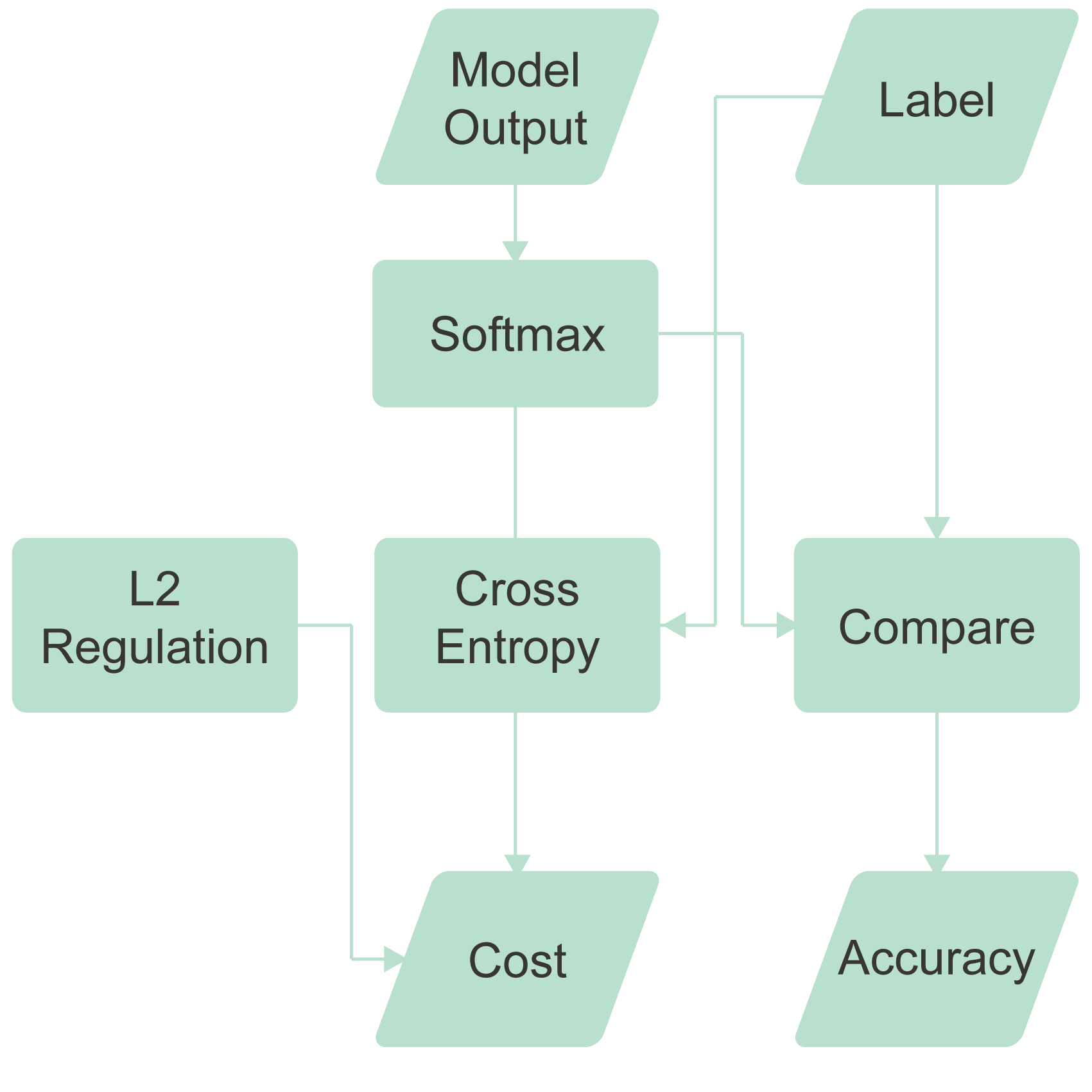#### Figure 3: Accuracy flow chart

We chose the cross-entropy as the network cost function because it performed well that the exclusion classification mission. Here this the formula of cross-entropy.

Suppose H is a cross-entropy function, y'i is the real label, and the yi the network prediction output, the cost is

$$H_{y'}(y) = - \sum_{i} y_{i}^{'}log(y_{i})$$

Parameters of the Neural Network were being optimized by Adam optimizer, which is a most commonly used ways to optimize the network.

After training, the model will be tested by independence test data, and the result was shown below.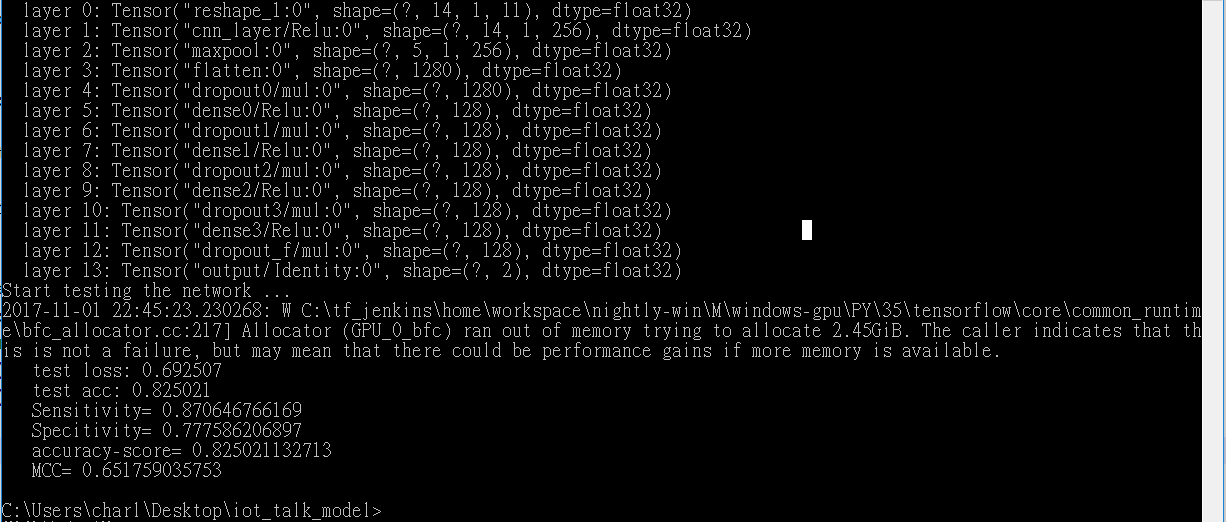#### Figure 4: Accuracy score = 82.5%

For the use in reality, the network would connect to our IoTtalk system, giving the user daily warning from the diseases happening.###### Spore Germination Modeling

To predict the occurrence of diseases more effectively and timely, we made the spore germination rate. According to the researchers, we first fitted out a linear equation for the spore germination rate base on the humidity and a cubic equation for the spore germination rate base on the temperature. By multiplying them, we get the general model for the spore germination. At last, the model was combined to IoTtalk to apply peptides into farmlands.

1. Fitted out the equation with researches and get the general spore germination rate model.
2. Conducted experiments as verification and determine the coefficients.
3. Result# Spore Germination Rate

Because we found out that the humidity and temperature affected the spore germination the most. Therefore, we wanted to build a general model for the spore germination rate based on temperature or humidity with different fungal species that can fit in every fungal species.

At first, we used the spore germination data on the research papers to fit out the functions.

## Spore germination based on temperature :

(1) Myce-liophthora thermophila's spore germination rate based on temperature

$$y = 0.0004x^3 - 0.0132x^2 + 4.0447x -24.746$$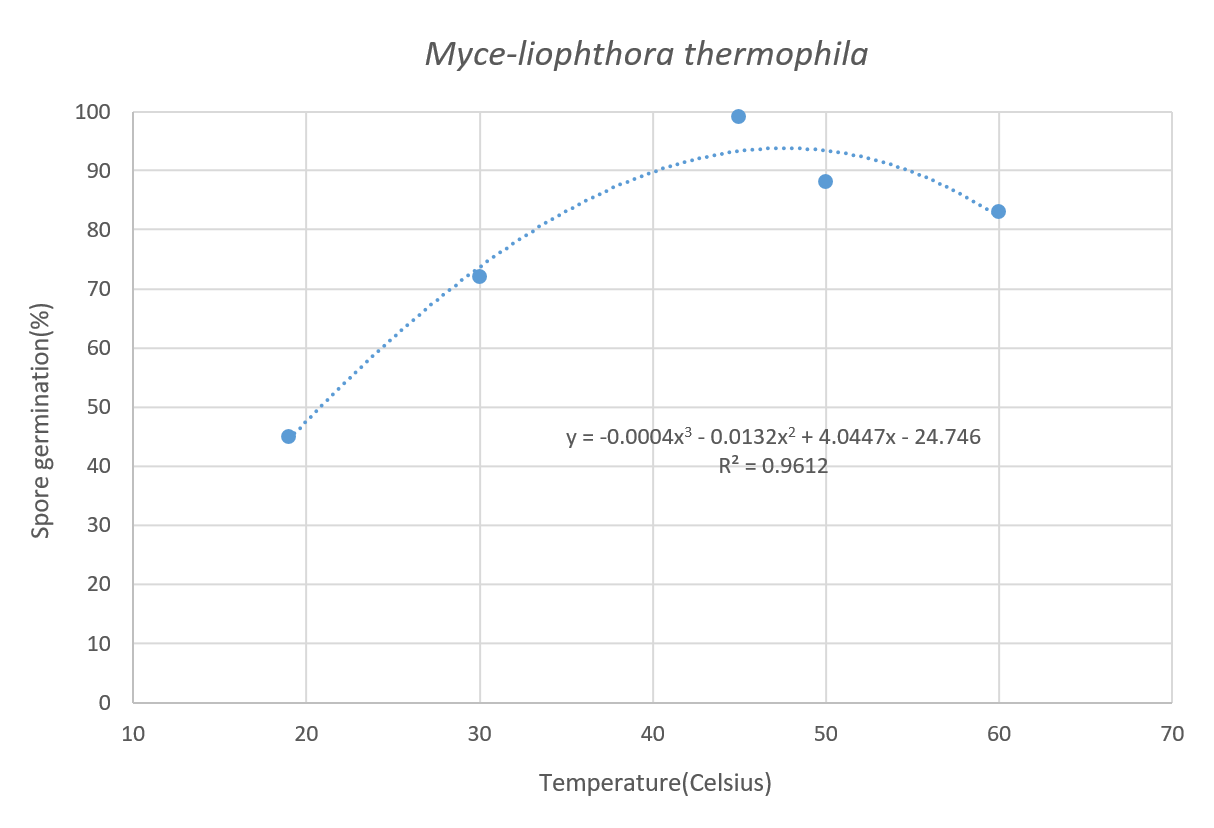#### Figure 5: Spore germination based on temperature.

(2) Aspergillus niger’s spore germination rate based on temperature:

$$y = 0.0326x^3 - 4.0593x^2 + 160.2x - 1943.7$$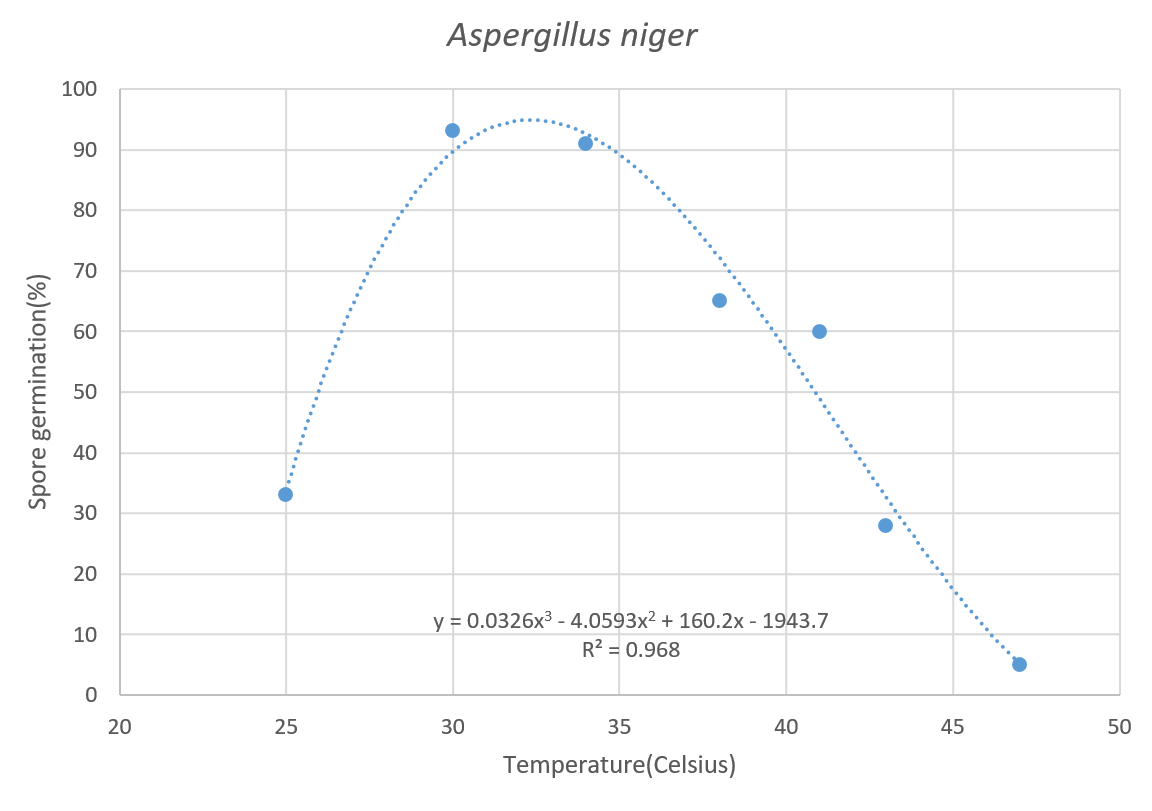#### Figure 6: Aspergillus niger’s spore germination rate based on temperature.

(3) P. oryzae’s spore germination rate based on temperature:

$$y = 0.06x^3 - 4.389x^2 + 106.86x - 774.66$$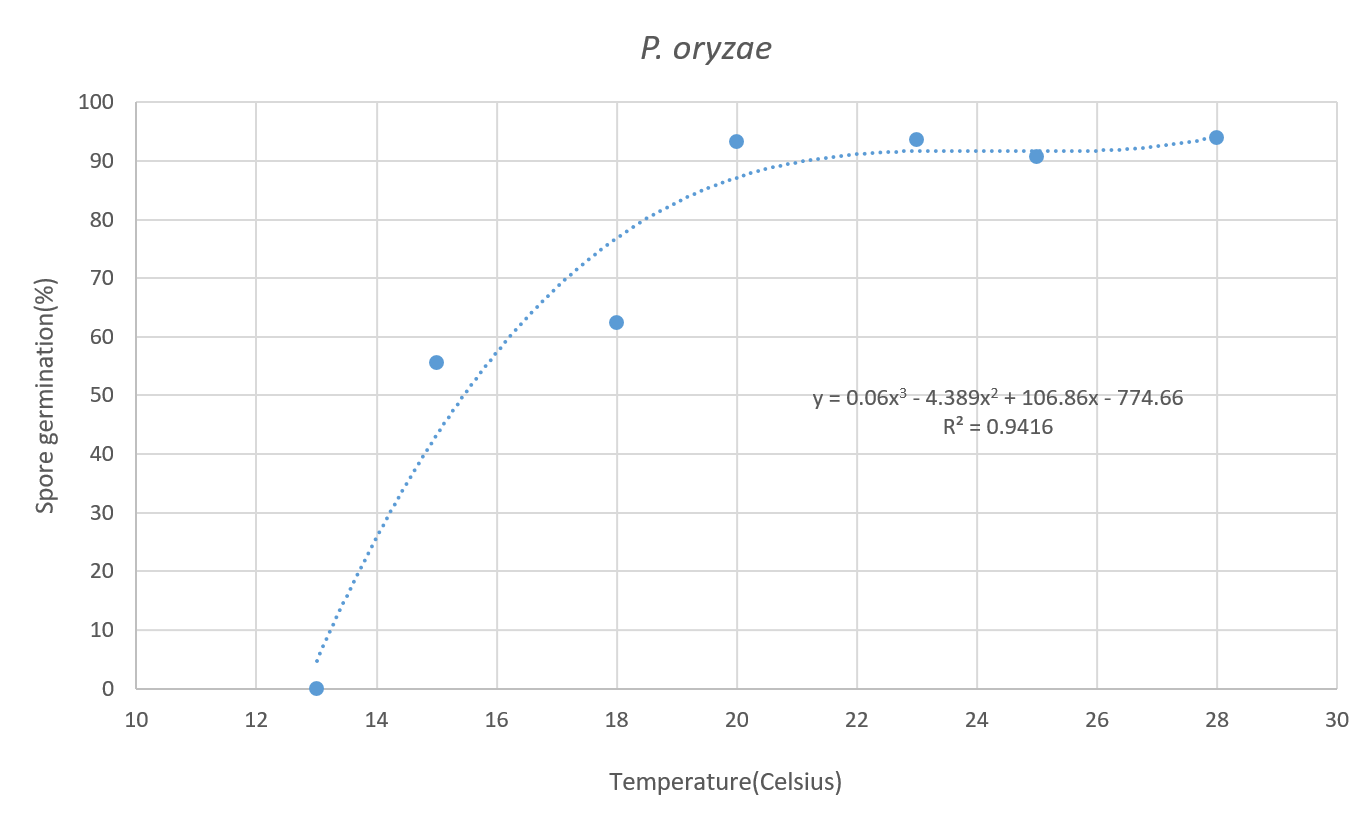#### Figure 7: P. oryzae’s spore germination rate based on temperature.

(4) Diplodia corticola’s spore germination rate based on temperature:

$$y = -0.0041x^3 + 0.0169x^2 + 7.1531x - 32.071$$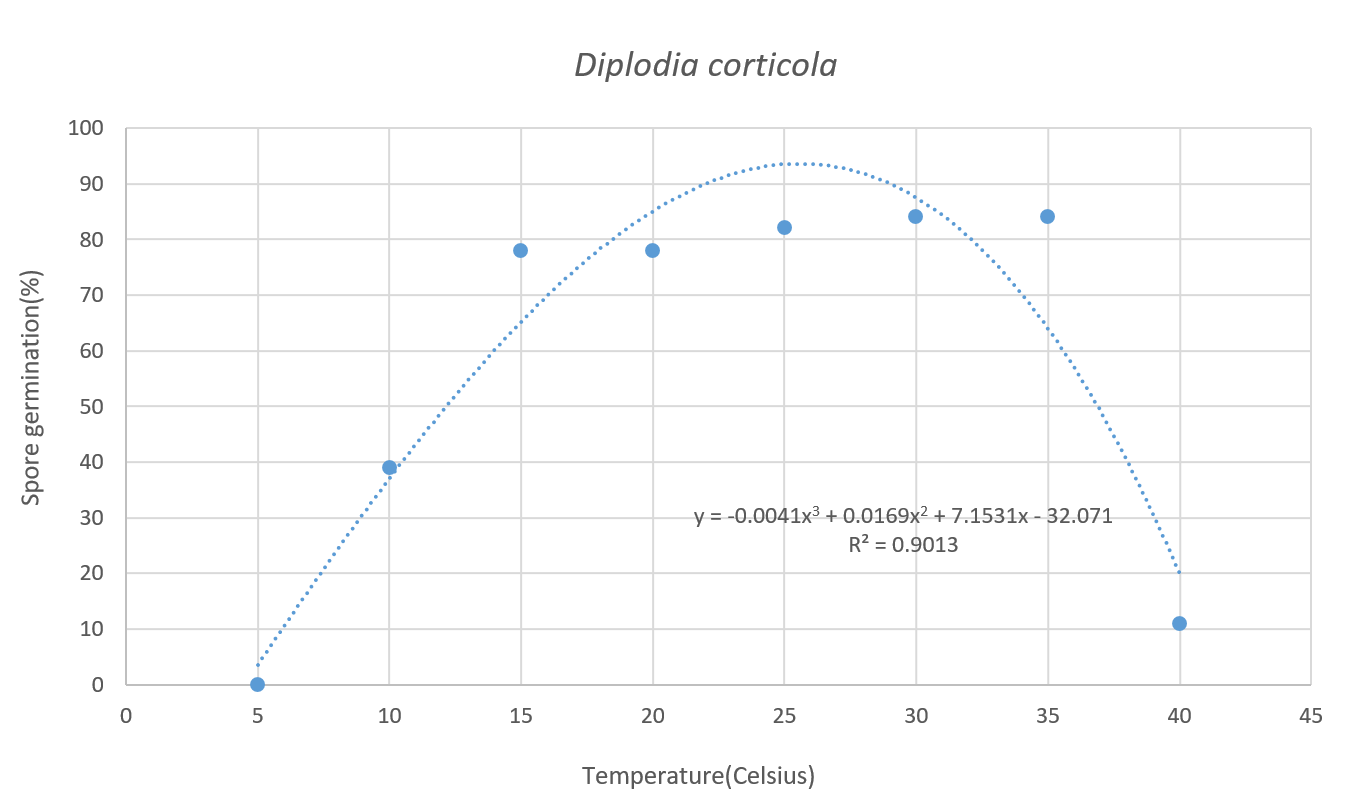#### Figure 8: Diplodia corticola’s spore germination rate based on temperature.

As results, we found out that the spore germination rate based on temperature can be fitted in a cubic equation

$$f_1(x) = ax^3 + bx^2 + cx + d$$

## Spore germination based on relative humidity:

(1) Aspergillus niger’s spore germination rate based on relative humidity:

$$y = 352.38x - 254.14$$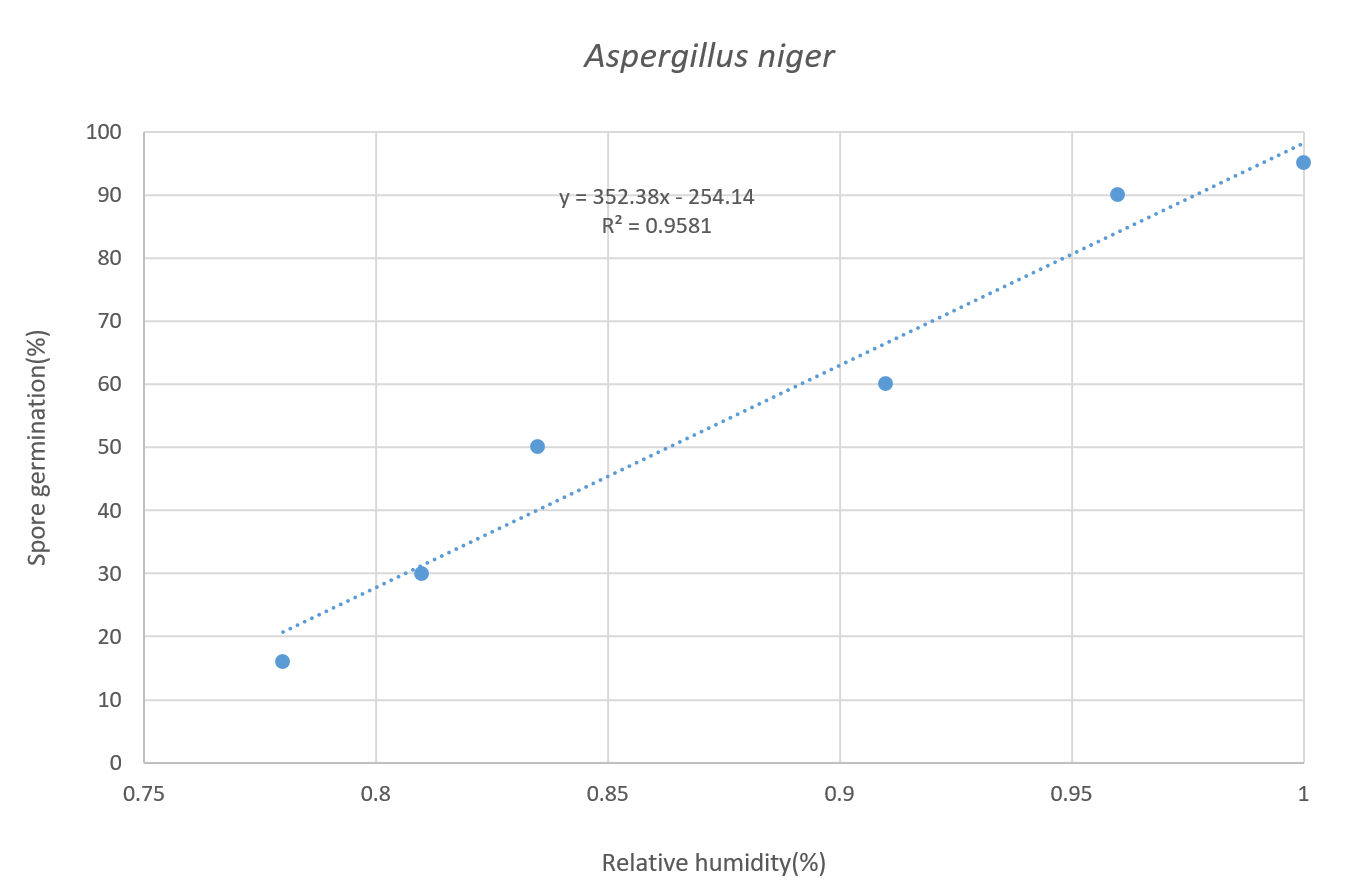#### Figure 9: Aspergillus niger’s spore germination rate based on relative humidity

(2) Pseudocercospora’s spore germination rate based on relative humidity:

$$y = 0.1071x - 10.165$$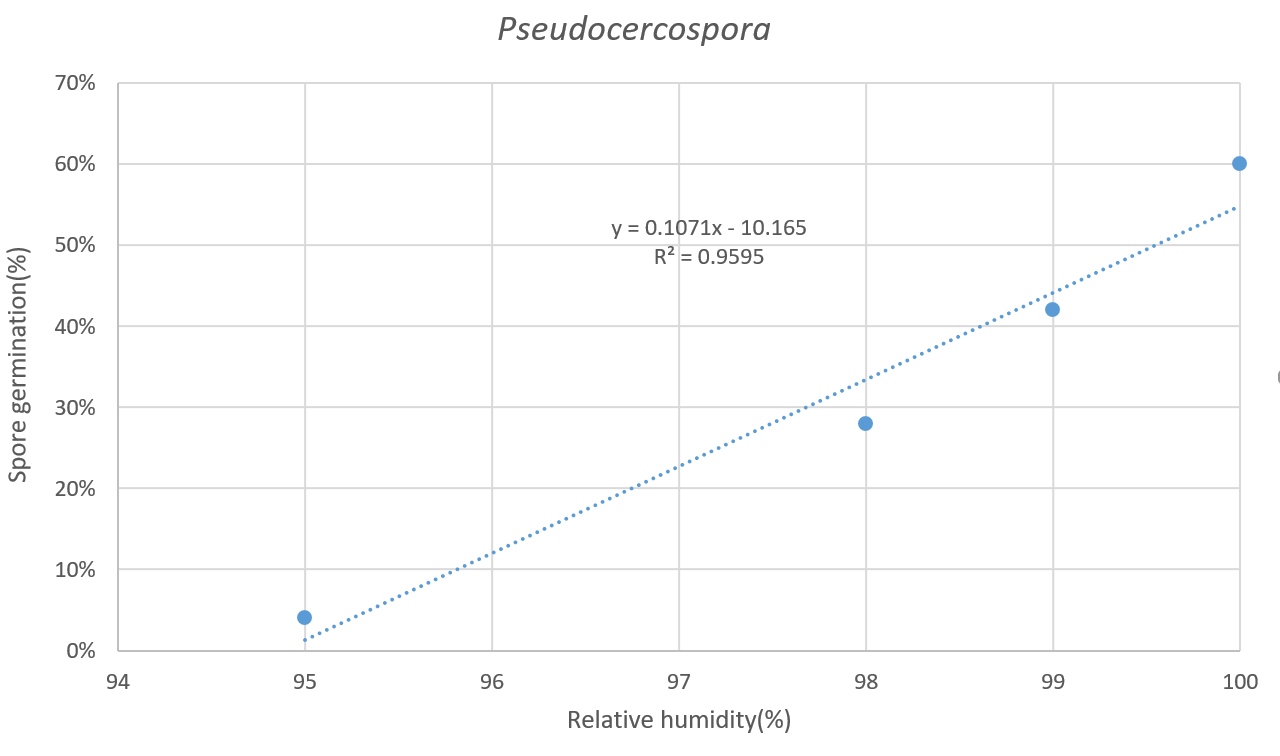#### Figure 10: Pseudocercospora’s spore germination rate based on relative humidity.

As results, we found out the spore germination rate based on the relative humidity in a linear equation.

$$f_2(x) = ax+b$$

## The General model of spore germination rate:

Later, we considered the two equations as independent events and multiplied them to form our general fungal spore germination model. Then we conducted experiments to determine the coefficients and verification.

$$f_1 \times f_2$$

In other words, Just need the temperature and humidity conditions, we can calculate the spore germination in that environment.

## Experiment Method:

The experiment part was divided into two part:

### Table 1: Overall experimental disign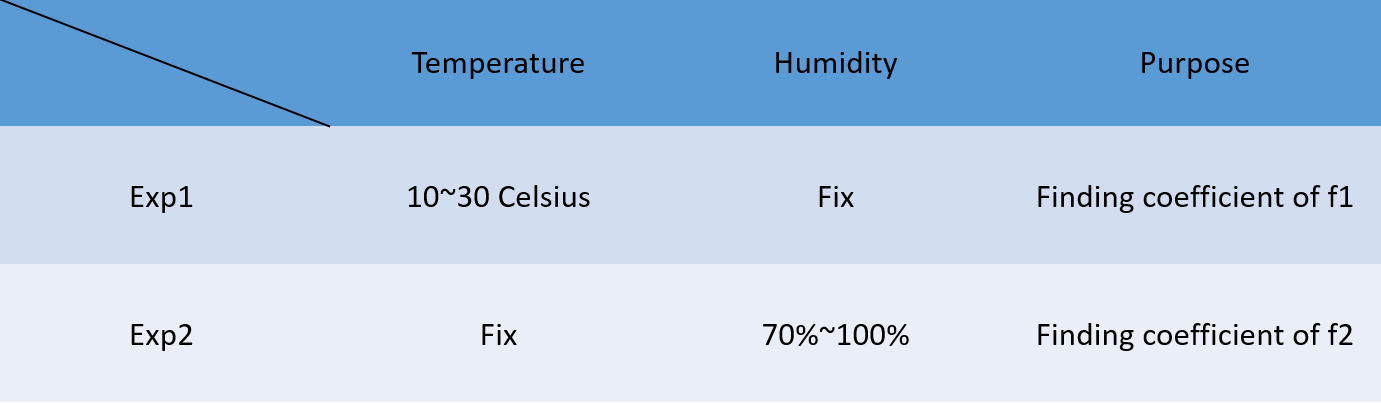(1) Fix humidity, change temperature:

Remove the spores from fungal plate by Distillation-Distillation H2O to made the spore suspension solution (2x105particles/mL) and 2% glucose solution mixing with equal volume, then put the solution in concave glass slides. Later, put it into temperature and humidity control box. The humidity was set at 100%, the temperature was set from 10 to 30 Celsius degrees and been tested once every 5 degrees.

(2) Fix temperature, change humidity:

Remove the spores from fungal plate by Distillation-Distillation H2O to made the spore suspension solution(2x105particles/mL)) and 2% glucose solution mixing with equal volume, then put the solution in concave glass slides. Later, put it into temperature and humidity control box. The temperature was fixed at 25 Celsius degrees, the humidity was set from 80% to 100% and been tested once every 5 percents.

(3)Independent event validation:

We compared the result of our formula with the value of the spore germination in the reality to verify temperature and relative humidity are independent event to spore germination. We randomly choose the conditions of temperature 23 and 13 Celsius, relative humidity 97% and 80%.

# Experiment Result:

The standard of spore germination

following pictures are the Illustration of whether the spore germinate or not.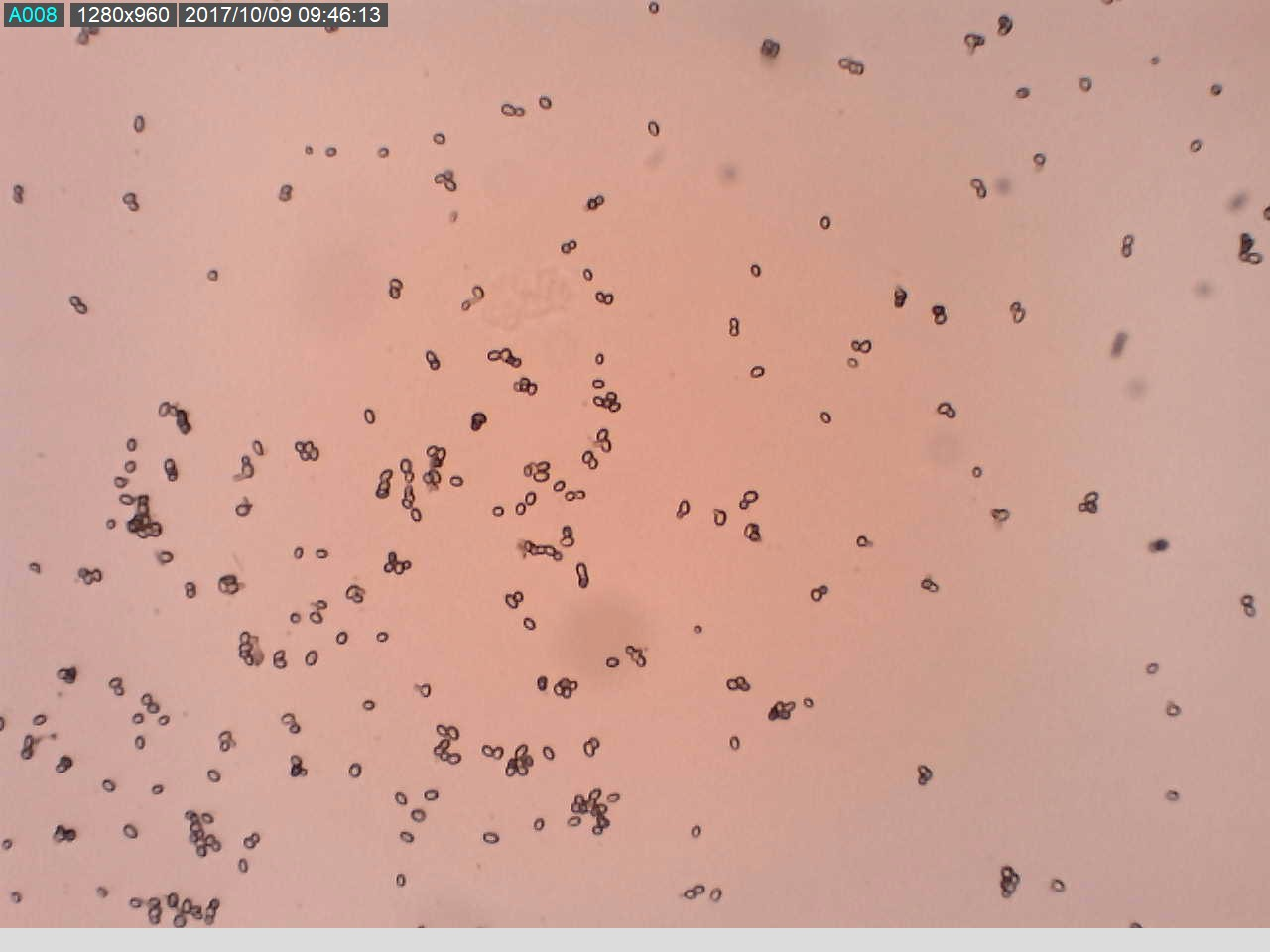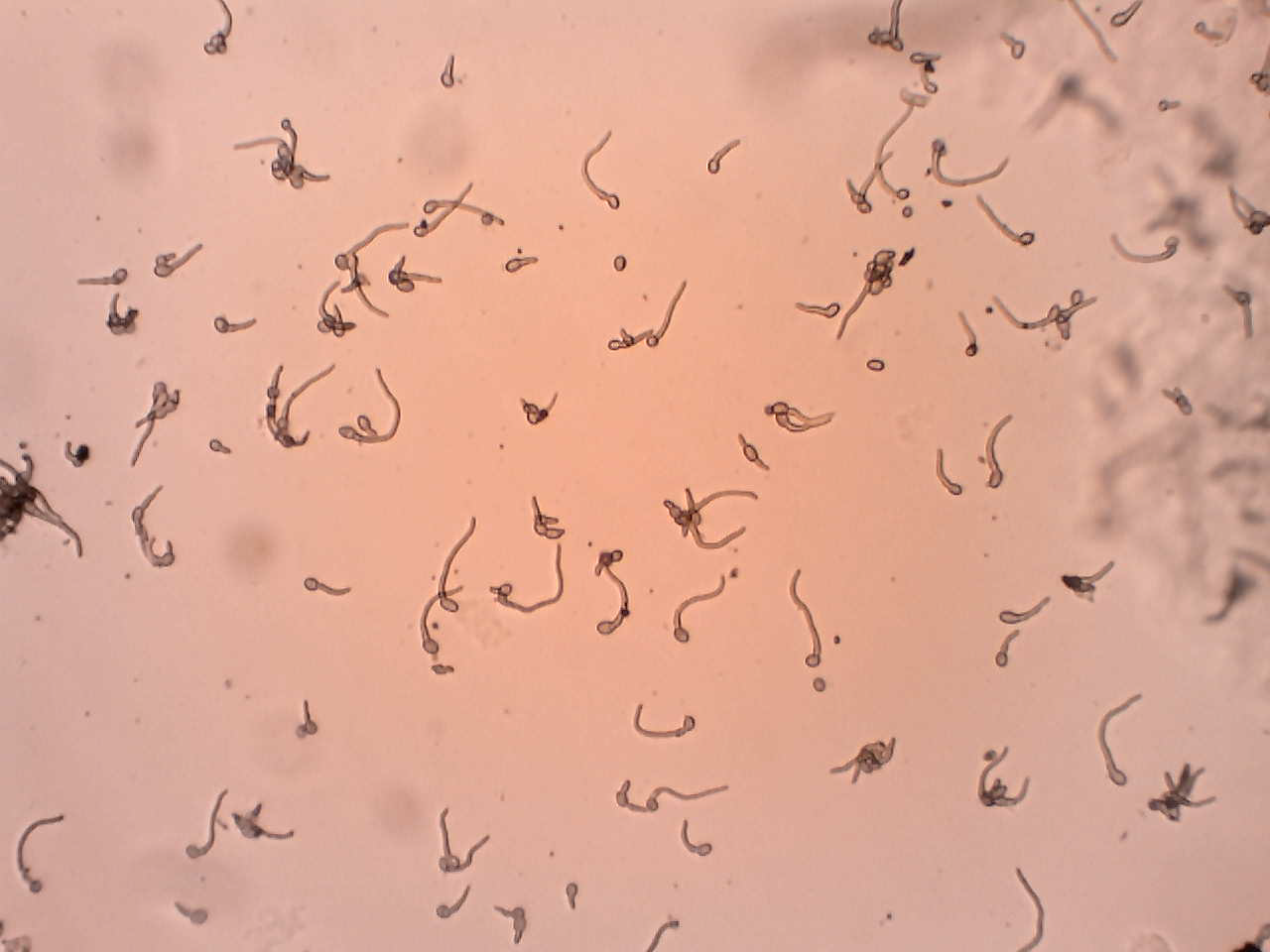#### Figure 12: (Right)25 Celsius degrees and 100% relative humidity (9 hours): Spore had germinated

(1) Fixed relative humidity in 100%:

The effective range of temperature is 10 to 30 Celsius degrees.

### Table 2: The spore germination rate of fixing the relative humidity and changing the temperature in different hours.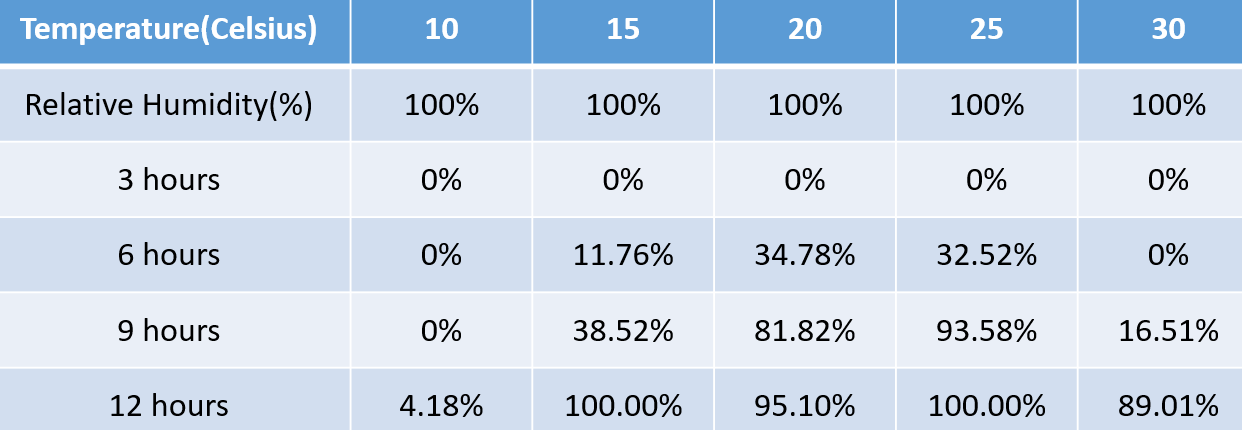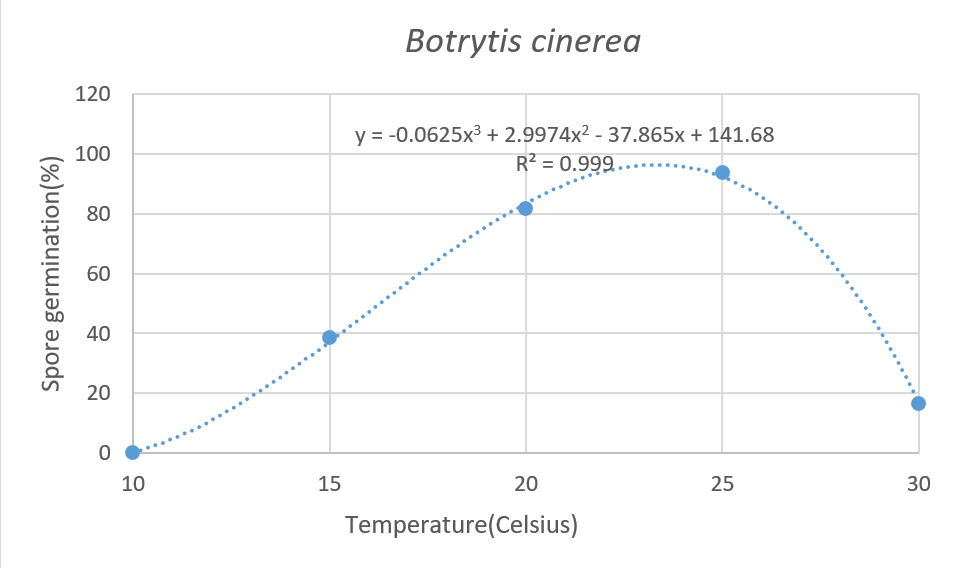#### Figure 13: Botrytis cinerea’s spore germination rate based on temperature.(9 hours)

Botrytis cinerea’s spore germination rate based on temperature:

$$f_1 = ( -0.0625x_1^3 + 2.9974x_1^2 - 37.865x_1 + 141.68)$$

(2) Fixed temperature in 20 Celsius

The effective range of relative humidity is 70%~100%, we use linear translation to let the spore germination in 100% relative humidity is also 100%.

### Table 3: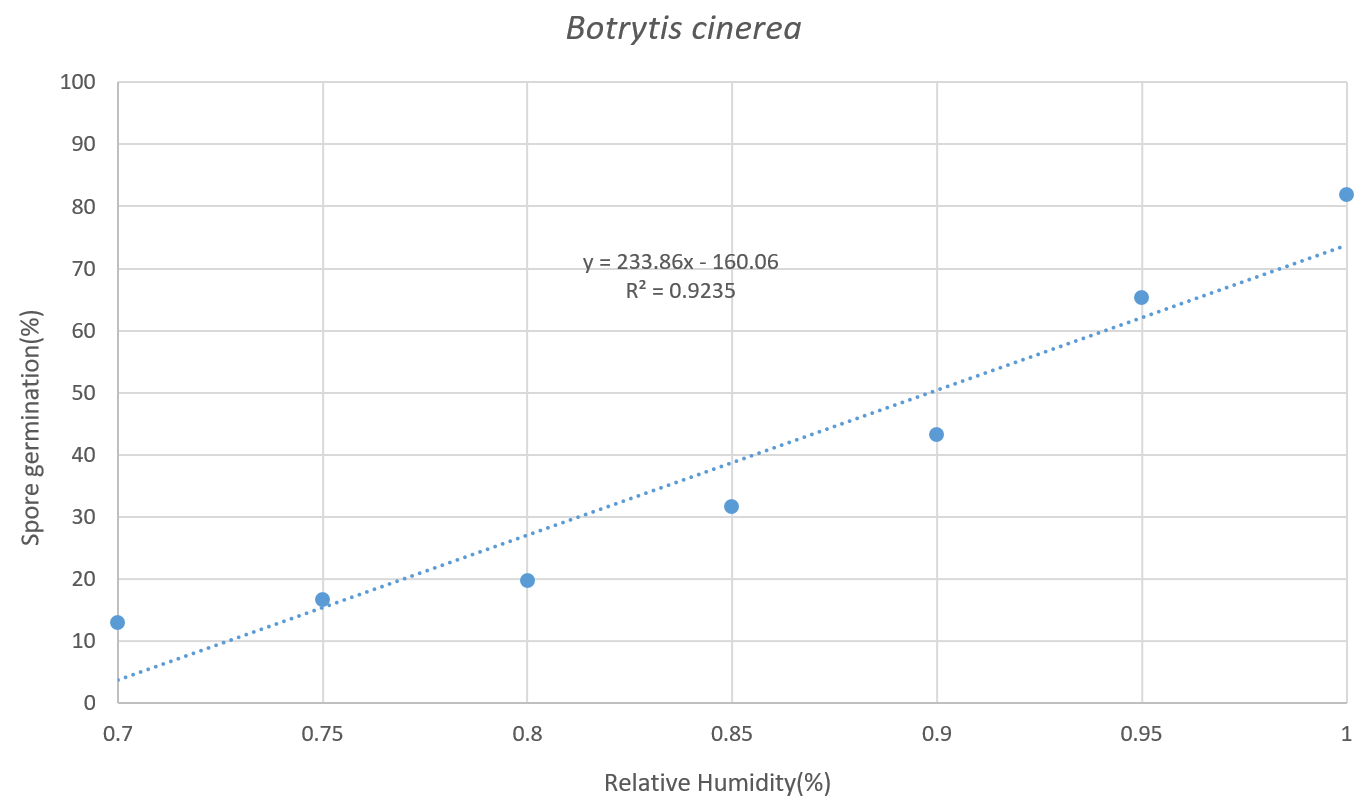#### Figure 14: Botrytis cinerea’s spore germination rate based on relative humidity(9 hours)

Botrytis cinerea’s spore germination rate based on relative humidity:

$$f_2 = 316.88x - 216.88$$

(3) Independent event validation

### Table 4: The results of our independent event validation.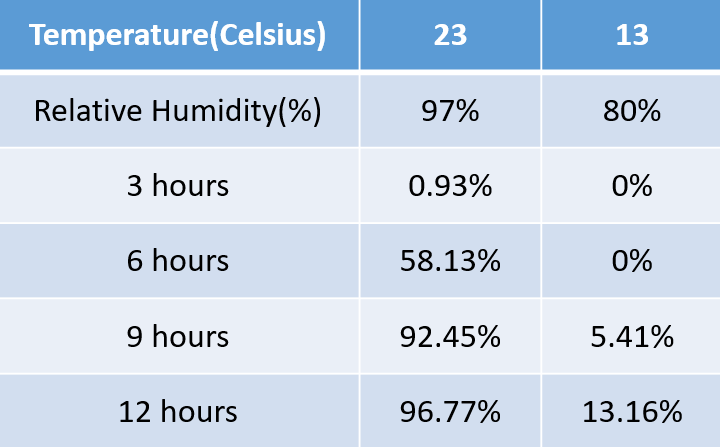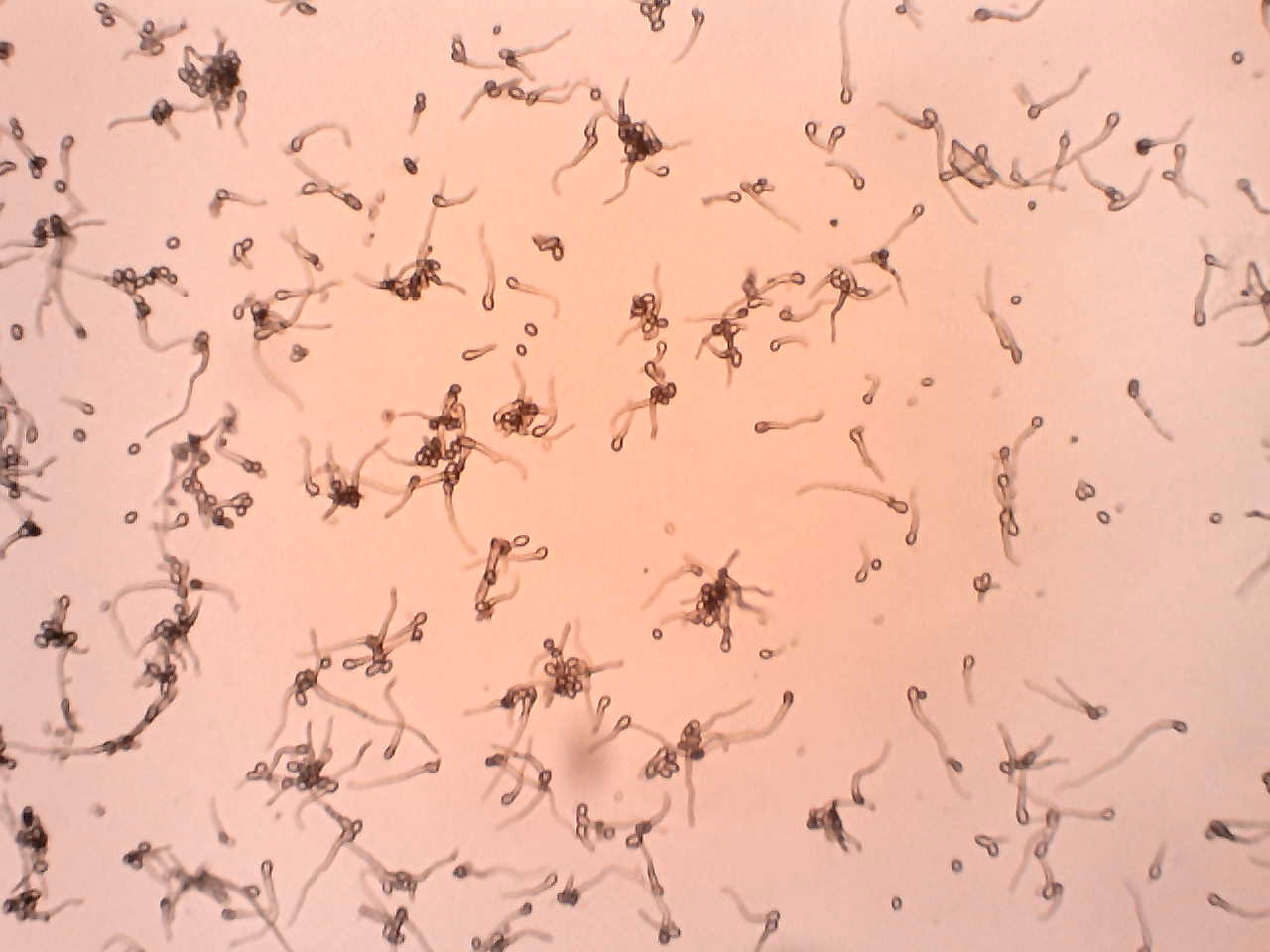#### Figure 15: The spore germination under the condition of 23 Celsius degrees and 97% relative humidity (9 hours)

In the conditions of 23 Celsius degrees and 97% relative humidity (9 hours) the spore germination rate by experiment is 92.45%. And according to our formula that the spore germination is 86.84%.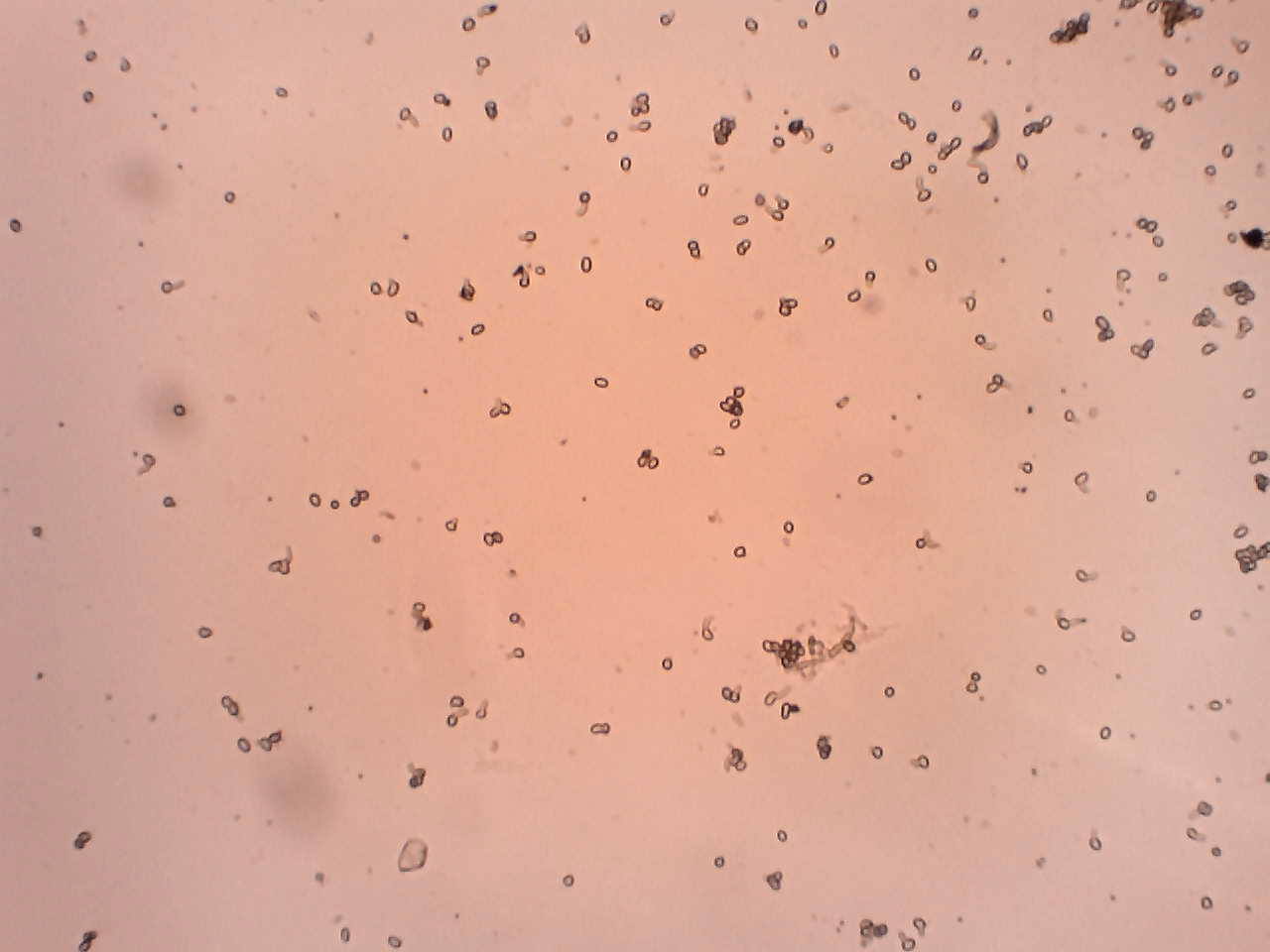#### Figure 16: The spore germination under the condition of 13 Celsius degrees and 80% relative humidity (9 hours).

In the condition of 13 Celsius degrees and 80% relative humidity (9 hours) the spore germination rate by experiment is 5.41%. And according to our formula that the spore germination is 6.84%.

The result can proof that our formula is quite precise.

### Table 5: The results of comparing the spore germination rate of our experiment and formula.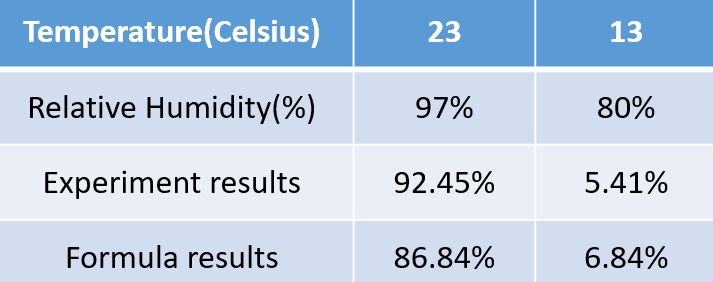## Conclusion:

Our final formula is:

$$f_1 \times f_2 = [(-0.0625x_1^3 + 2.9974x_1^2 - 37.865x_1 + 141.8)\div 100]\times [(316.88x_2 - 216.88)\div 100]$$

where x1 is temperature and x2 is relative humidity.

According our experiment result, we prove that temperature and relative humidity is an independent event and our formula is quite precise.

# Combined with IoTtalk

IoT immediately detects temperature and humidity (per hour detection), and we put the temperature and humidity condition that IoT detected into the formula we developed to calculate spore germination. If the spore germination rate exceeds a certain value, we will inform the user that the disease may occur.

If both of the predicted results are above the threshold, the user will be advised to spray the pesticide. The data sent back to IoT system simultaneously and the user can decide whether to automatically spray.

When the germination rate exceeds the threshold, we will advise the user to spray the corresponding peptide.

# Reference

H. HASSOUNI1, et al. COMPARATIVE SPORE GERMINATION OF FILAMENTOUS FUNGI ON SOLID STATE FERMENTATION UNDER DIFFERENT CULTURE CONDITIONS. 2006

Reference:Shivani R, Top 6 Factors Influencing Disease Cycle of Rice | Plant Disease

Reference:José R. Úrbez-Torres, Effect of Temperature on Conidial Germination of Botryosphaeriaceae Species Infecting Grapevines, 2010

Awad M. Abdel-Rahim, Hassan A. Arbab, Factors affecting spore germination in Aspergillus niger, 1985

L. H. Jacome, W. Schuh, and R. E. Stevenson Effect of Temperature and Relative Humidity on Germination and Germ Tube Development of Mycosphaerella fijiensis var. difformis, 1991Untitled Document